# 深度学习里常用激活函数，目标函数

### 激活函数

（0）阶跃激活函数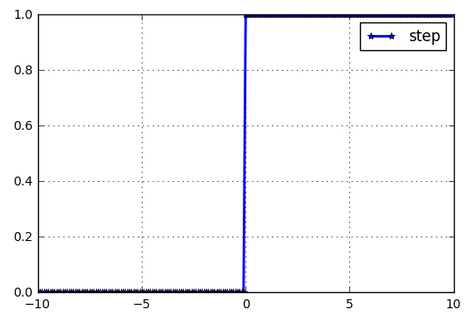（1）sigmoid激活函数，tanh激活函数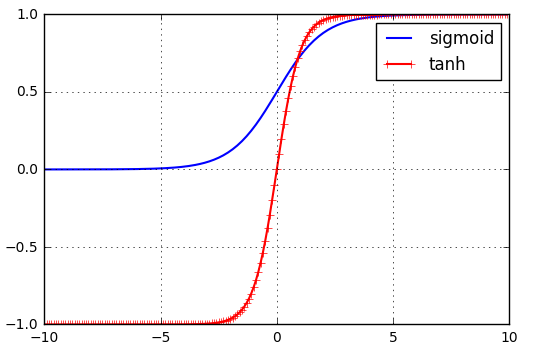（2）ReLU激活函数，Leaky-ReLU激活函数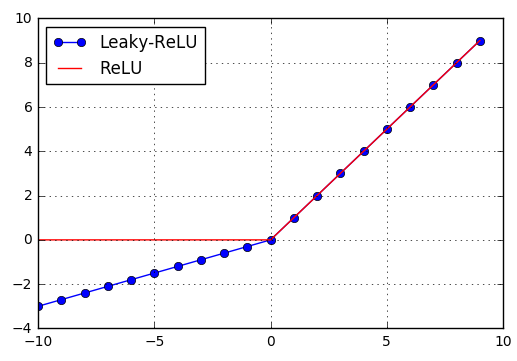（3）softmax激活函数

softmax(xi)=exij=1nexj$softmax(x_i)=\frac{e^{x_i}}{\sum\limits_{j=1}^{n}e^{x_{j}}}$

### 目标函数

#### （1）二次代价函数

C=12ni=1n||yia(xi)||2$C=\frac{1}{2n}\sum\limits_{i=1}^{n}||y_i-a(x_i)||^2$
a(xi)=σ(zi)=σ(wTxi+b)=σ(j=1mwjxi,j+b)$其中a(x_i)=\sigma(z_i)=\sigma(w^{T}x_i+b)=\sigma(\sum\limits_{j=1}^{m}w_jx_{i,j}+b)$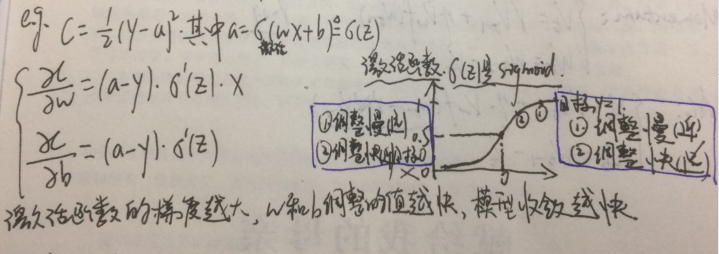#### （2）交叉熵代价函数

C=1ni=1n[yiloga(xi)+(1yi)log(1a(xi))]$C=-\frac{1}{n}\sum\limits_{i=1}^{n}[y_iloga(x_i)+(1-y_i)log(1-a(x_i))]$

xi=(xi,1,xi,2,,xi,m)T$x_i=(x_{i,1},x_{i,2},\cdots,x_{i,m})^T$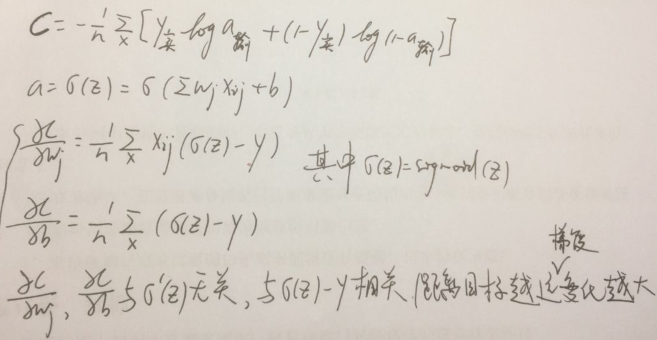#### （3）对数似然代价函数